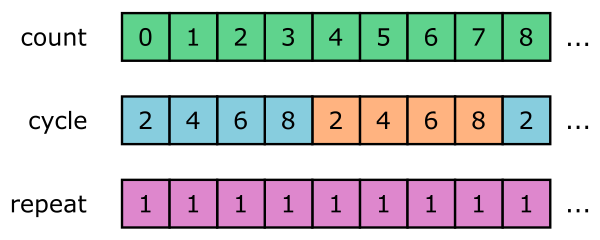# itertools module - infinite iterators

By Martin McBride, 2021-10-11
Tags: count cycle repeat itertools python standard library
Categories: python standard libraryThe itertools module provides several infinite iterators. These will return a potentially infinite number of values.

The iterators in this section are:

• count
• cycle
• repeat

## count

The count function returns an iterator that creates values 0, 1, 2 ... and keeps going forever. It works a bit like the range function, except that it has no upper limit. Here is an example:

for i in itertools.count():
print(i)


This loop will print 0, 1, 2 and so on. Since the count function will carry on forever, you will normally need some kind of break statement in the loop to force it to stop at some point.

The count function has two optional parameters, start which sets the start value (default 0), and step which sets the step value (default 1).

One interesting use of count is with the zip function, to combine two or more iterators. Here is an example:

k = ['a', 'b', 'c']

for i, c in zip(itertools.count(2, 3), k):
print(i, c)


This combines a count that starts from 2 in steps of 3, and the list k. Since zip terminates when the shortest sequence ends, the loop will only run 3 times (because k has length 3). Here is what it prints:

2 a
5 b
8 c


This is a bit like the enumerate function, but with the option to have a step value.

## cycle

The cycle function creates an infinite iterator based on an iterable. When the iterable is used up, cycle will repeat the values, indefinitely. For example:

k = [2, 4, 6, 8]

for i in itertools.cycle(k):
print(i)/programming-techniques/functional-programming/iterators/


This will print 2, 4, 6, 8, 2, 4, 6, 8 ... forever.

## repeat

repeat is similar to cycle, but it only has one value that it repeats over and over. It will continue indefinitely unless the optional times parameter is supplied:

for i in itertools.repeat(5, times=3):
print(i)


This prints 5, 5, 5 because the times parameter is set to 3. If you omit this parameter, it will repeat forever.

Here is a diagram of count, cycle and repeat:### Potential problem with infinite iterators

The following code can cause problems:

k = list(itertools.count())


The problem here is that list wants to create a list containing every element in the iterator returned by itertools.count(). To do this it will attempt to loop through every element in the iterator and store it in memory. But since the list is infinite, this process will never complete successfully.

list will go into a tight, infinite loop, allocating itself huge amounts of memory.

Depending on how well your operating system handles this, you will probably encounter some degree of instability or slow down. Worst case the UI might become so unresponsive that you are unable to access the system tools to kill the program.

This same caveat applies to any functions that attempt to consume the whole iterable before returning. This includes certain built-in functions (such as sum) that need to consume all the data before returning an answer, and also the combinatoric itertools functions described below.